## ICSE Maths Previous Year Question Paper 2011 Solved for Class 10

ICSE Paper 2011
MATHEMATICS

(Two hours and a half)
Answers to this Paper must be written on the paper provided separately.
You will not be allowed to write during the first 15 minutes.
This time is to be spent in reading the question paper.
The time given at the head of this Paper is the time allowed for writing the answers.
Attempt all questions from Section A and any four questions from Section B.
All working, including rough work, must be clearly shown and must be done on the same sheet as the rest of the answer. Omission of essential working will result in the loss of marks.
The intended marks for questions or parts of questions are given in brackets [ ].
Mathematical tables are provided.

SECTION-A  (40 Marks)
(Attempt all questions from this Section)

Question 1:
(a) Find the value of ‘k’ if (x – 2) is a factor of x3 + 2x – kx + 10?(c) Mr. Kumar borrowed Rs. 25,000 for two years. The rate of interest for the two successive years are 8% and 10% respectively. If he repays Rs. 6,200 at the end of the first year, find the outstanding amount at the end of the second year. 

Solution:
(a)(b)(c)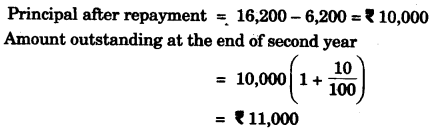Question 2:
(a) From a pack of 52 playing cards all cards whose numbers are multiples of 3 are removed. A card is now drawn at random.
What is the probability that the card drawn is:
(i) a face card (King, Jack or Queen)
(ii) an even-numbered red card?      
(b) Solve the following equation:
x – 18/x = 6. Give your answer correct to two significant figures. 
(c) In the given figure O is the centre of the circle. Tangents at A and B meet at C. If ∠AOC = 30°, find
(i) ∠BCO
(ii) ∠AOB
(iii) ∠APB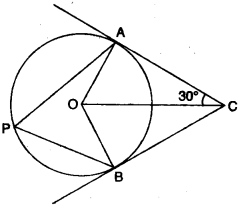Solution:
(a) Number of cards which are multiples of 3 = 12
Cards left in the pack = 40
Number of face cards = 12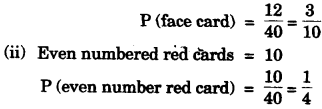(b)(c)Question 3:
(a)
Ahmed has a recurring deposit account in a bank. He deposits Rs. 2,500 per month for 2 years. If he gets Rs. 66,250 at the time of maturity, find
(i) The interest paid by the bank.
(ii) The rate of interest.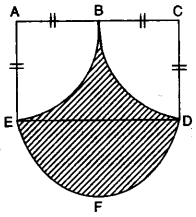(b) Calculate the area of the shaded region, if the diameter of the semi-circle is equal to 14 cm. Take π = 22/7 
(c) ABC is a triangle and G(4, 3) is the centroid of the triangle. If A = (1, 3), B = (4, b) and C = (a, 1), find ‘a’ and ‘b’. Find the length of side BC. 
Solution:
(a)(b)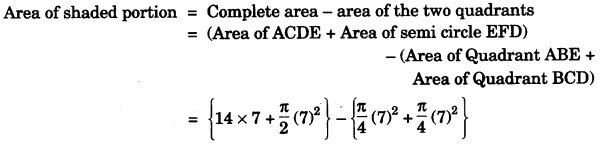(c)Question 4:
(a) Solve the following inequation and represent the solution set on the number line 2x – 5 ≤ 5x + 4 < 11, where x ∈ 1.  
(b) Evaluate without using trigonometric tables:(c) A Mathematics aptitude test of 50 students was recorded as follows:

 Marks 50-60 60-70 70-80 80-90 90-100 No. of students 4 8 14 19 5

Draw a histogram for the above data using a graph paper and locate the mode. 

Solution:
(a)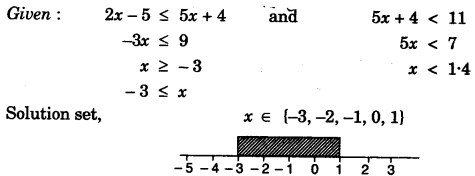(b)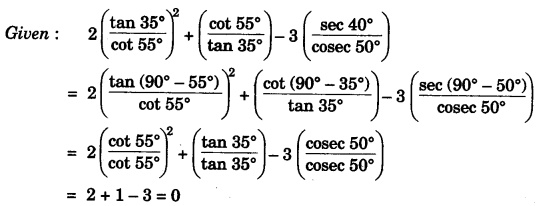(c)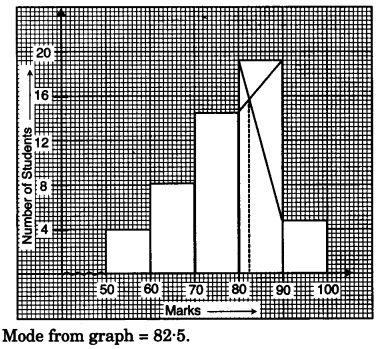SECTION-B  (40 Marks)
(Attempt any four questions from this Section)

Question 5:
(a) A manufacturer sells a washing machine to a wholesaler for Rs. 15,000. The wholesaler sells it to a trader at a profit of Rs. 1,200 and the trader in turn sells it to a consumer at a profit of Rs. 1,800. If the rate of VAT is 8% find:
(i) The amount of VAT received by the State Government on the sale of this machine from the manufacturer and the wholesaler.
(ii) The amount that the consumer pays for the machine. 
(b) A solid cone of radius 5 cm and height 8 cm is melted and made into small spheres of radius 0.5 cm. Find the number of spheres formed. 
(c) ABCD is a parallelogram where A(x, y), B(5, 8), C(4, 7) and D(2, -4). Find
(i) Coordinates of A
(ii) Equation of diagonal BD. 

Solution:
(a)(b)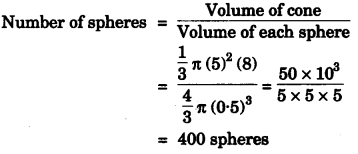(c)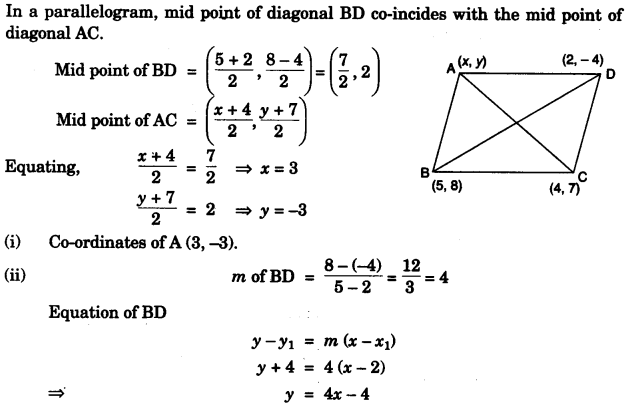Question 6:
(a) Use a graph paper to answer the following questions. (Take 1 cm = 1 unit on both axes).
(i) Plot A (4, 4), B (4, -6) and C (8, 0), the vertices of a triangle ABC.
(ii) Reflect ABC on the y-axis and name it as A’B’C’.
(iii) Write the coordinates of the image A’, B’ and C’.
(iv) Give a geometrical name for the figure AA’C’B’BC.
(v) Identify the line of symmetry ofAA’C’B’BC.       
(b) Mr. Choudhury opened a Saving’s-Bank Account at State Bank of India on 1st April 2007. The entries of one year as shown in his pass book are given below:

 Date Particulars Withdrawals(in Rs.) Deposits(in Rs.) Balance(in Rs.) 1st April 2007 By Cash — 8550.00 8550.00 12th April 2007 To Self 1200.00 — 7350.00 24th April 2007 By Cash — 4550.00 11900.00 8th July 2007 By Cheque — 1500.00 13400.00 10th Sept. 2007 By Cheque — 3500.00 16900.00 17th Sept. 2007 To Cheque 2500.00 — 14400.00 11th Oct. 2007 By Cash — 800.00 15200.00 6th Jan. 2008 To Self 2000.00 — 13200.00 9th March 2008 By Cheque — 950.00 14150.00

If the bank pays interest at the rate of 5% per annum, find the interest paid on 1st April, 2008. Give your answer correct to the nearest rupee. 

Solution:
(a)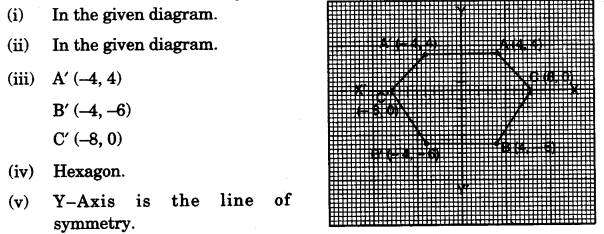(b)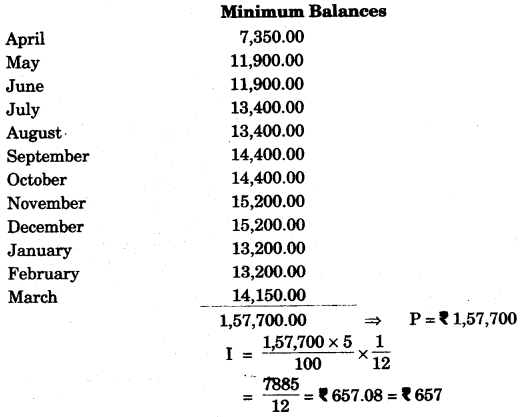Question 7:
(a) Using componendo and dividendo, find the value of x.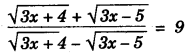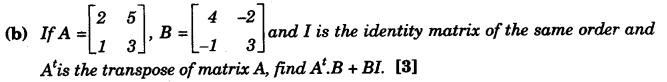(c)Solution:
(a)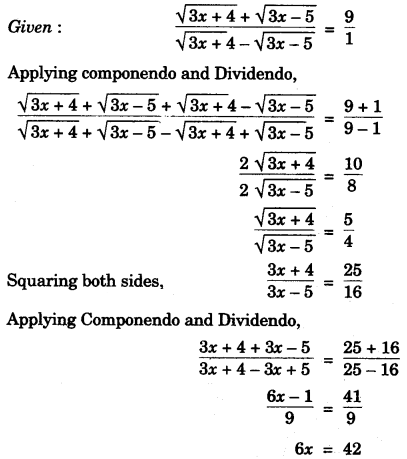(b)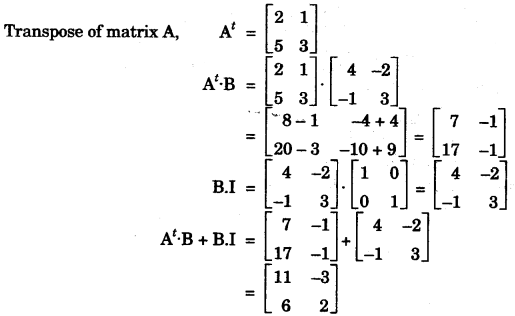(c)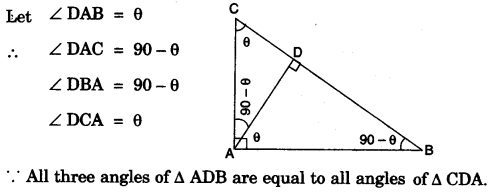Question 8:
(a) (i) Using step-deviation method, calculate the mean marks of the following distribution.
(ii) State the modal class: 

 Class Interval 50-55 55-60 60-65 65-70 70-75 75-80 80-85 85-90 Frequency 5 20 10 10 9 6 12 8

(b) Marks obtained by 200 students in an examination are given below:

 Maries 0-10 10-20 20-30 30-40 40-50 50-60 60-70 70-80 80-90 90-100 No. of Students 5 11 10 20 28 37 40 29 14 6

Draw an ogive for the given distribution taking 2 cm = 10 marks on one axis and 2 cm = 20 students on the other axis. Using the graph, determine
(i) The median marks.
(ii) The number of students who failed if minimum marks required to pass is 40.
(iii) If scoring 85 and more marks is considered as grade one, find the number of students who secured grade on in the examination. 

Solution:
(a)(b)Question 9:
(a) Mr. Parekh invested Rs. 52,000 on 100 shares at a discount of Rs. 20 paying 8% dividend. At the end of one year he sells the shares at a premium of Rs. 20. Find
(i) The annual dividend.
(ii) The profit earned including his dividend. 
(b) Draw a circle of radius 3.5 cm. Mark a point P outside the circle at a distance of 6 cm. from the centre. Construct two tangents from P to the given circle. Measure and write down the length of one tangent. 
(c) Prove that (cosec A – sin A) (sec A – cos A ) sec2 A = tan A. 

Solution:
(a) Investment = Rs. 52,000
N.V = Rs. 100
M.V. of one share = Rs. (100 – 20) = Rs. 80
Dividend = 8%
No. of shares = Investment/MV(b)(c)Question 10:
(a) 6 is the mean proportion between two numbers x and y and 48 is the third proportional of x and y. Find the numbers. 
(b) In what period of time will Rs. 12,000 yield Rs. 3,972 as compound interest at 10% per annum, if compounded on an yearly basis? 
(c) A man observes the angle of elevation of the top of a building to be 30°. He walks towards it in a horizontal line through its base. On covering 60 m the angle of elevation changes to 60°. Find the height of the building correct to the nearest metre. 

Solution:
(a)(b)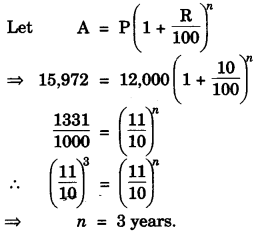(c)Question 11:
(a) ABC is a triangle with AB = 10 cm, BC = 8 cm and AC = 6 cm (not drawn to scale). Three circles are drawn touching each other with the vertices as their centres. Find the radii of the three circles.(b)
Rs. 480 is divided equally among ‘x’ children. If the number of children was 20 more then each would have got Rs. 12 less. Find ‘x’. 
(c) Given equation of line L1 is y = 4.(i) Write the slope of line L2 if L2 is the bisector of angle O.
(ii) Write the co-ordinates of point P.
(iii) Find the equation of L2.   

Solution:
(a) Let the three radii be x, y, z respectively.
x + y = 10          ……… (1)
y + z = 8            ……… (2)
x + z = 6           ……… (3)
Adding equation’s (1), (2) and (3), 2x + 2y + 2z = 24
x + y + z = 12     ……… (4)
Subtracting each equation (1), (2) and (3) from equation (4), we get
z = 2 cm, x = 4 cm, y = 6 cm.
(b)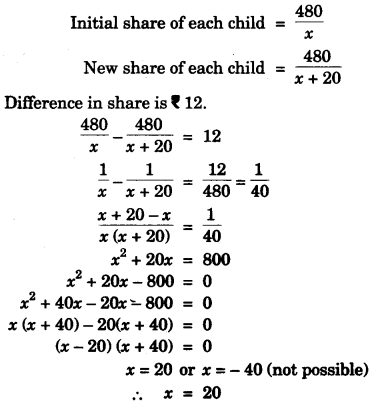(c)